## Wednesday, March 31, 2010

### 808

808 = 23 x 101.

808 has a representation as a sum of two squares: 808 = 182 + 222.

The first 808 digits of 808! form a prime number.

808 is a strobogrammatic number. It is the same number when rotated 180 degrees on an axis perpendicular to the plane on which the number is written.

The 808th Fibonacci number plus and minus 808 results in (132)-digit primes.The Roland TR-808 was one of the first programmable drum machines, introduced in early 1980.

## Tuesday, March 30, 2010

### 314

314 = 2 x 157.

314 has a representation as a sum of two squares: 314 = 52 + 172.

314 is the smallest number that can be written as the sum of three positive squares in six ways: 314 = 172 + 42 + 32 = 162 + 72 + 32 = 152 + 82 + 52 = 132 + 92 + 82 = 132 + 122 + 12 = 132 + 92 + 82.

314 is 222 in base 12.

314 is the only number that together with its sixth power, 958468597212736, has each digit from 1 to 9 occurring exactly twice.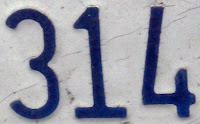314 is the telephone area code for St. Louis, Missouri.

## Monday, March 29, 2010

### 2265

2265 = 3 x 5 x 151. It has exactly three distinct palindromic prime factors.

The sum of the digits of 2265 equals the sum of the digits of its prime factors: 2 + 2 + 6 + 5 = 3 + 5 + 1 + 5 + 1 = 15.

The product of the digits of 2265 is eight times the sum of the digits: 2 x 2 x 6 x 5 = 8(2 + 2 + 6 + 5) = 120.

2265 is 14253 in base 6. It uses each positive digit just once.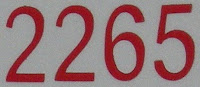2265 is a year in which January 1 falls on a Sunday.

## Friday, March 26, 2010

### 3531

3531 = 3 x 11 x 107.

3531 is a composite number whose three prime factors are distinct in length.

3531 is 24203 in base 6 and 13203 in base 7.

3531 is a multiple of 11 having only odd digits.

3531 is the number of sums payable using exactly 39 banknotes of denominations 1, 5, 10, 20, 50, and 100. For n = 1, there are precisely six payable sums.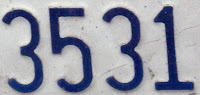The Lego 3531 set was a kit for building the Znap Tri-bike.

## Thursday, March 25, 2010

### 9002

9002 = 2 x 7 x 643.

9002 = 32 x 103 + 2.

9002 is a value of n such that n + 7 and n(n + 7) are palindromes: 9009 and 81099018.

9002 is a number n such that n(n + 7) is a palindrome: 9002(9009) = 81099018.ISO 9002 was an international standard to used to certify manufacturing quality.

## Wednesday, March 24, 2010

### 774

774 = 2 x 32 x 43.

774 is 3330 in base 6 and 1050 in base 9.

The sum of the digits of 774 is six times the number of digits: 7 + 7 + 4 = 18 = 6 x 3.

774 is the sum of twelve positive fifth powers: 744 = 35 + 35 + 25 + 25 + 25 + 25 + 25 + 25 + 25 + 25 + 15 + 15.774 Armor is an asteroid orbiting the Sun.

## Tuesday, March 23, 2010

### 986

986 = 2 x 17 x 29.

986 has two representations as a sum of two squares: 986 = 52 + 312 = 192 + 252.

986 is a strobogrammatic number. It is a number that is the same whether it is viewed normally or upside down.

986 = 19 + 28 + 36.

986 is 12421 in base 5.NGC 986 is a galaxy in the constellation Fornax.

## Monday, March 22, 2010

### 803

803 = 11 x 73.

803 is the sum of three consecutive prime numbers: 803 = 263 + 269 + 271. It is also the sum of nine consecutive primes: 803 = 71 + 73 + 79 + 83 + 89 + 97 + 101 + 103 + 107.

803 is 30203 in base 4, 323 in base 16, and 203 in base 20.

803 is a divisor of 99999999.Telephone area code 803 covers southern South Carolina.

## Friday, March 19, 2010

### 593

593 is a prime number. It is a Sophie Germain prime because 2 x 593 + 1 = 1187 is also prime.

593 has a representation as a sum of two squares: 593 = 82 + 232.

593 is the hypotenuse of a primitive Pythagorean triple: 5932 = 3682 + 4652.

593 = 92 + 29 = 32*2 + 23*3.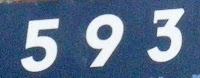593 is the number on the conning tower of the submarine USS Wayne in the movie The Spy Who Loved Me.

## Thursday, March 18, 2010

### 388

388 = 22 x 97.

388 has a representation as a sum of two squares: 388 = 82 + 182.

The number 388 followed by 388 sevens is a prime number.

388 is the maximum value of n so that there exist 6 denominations of stamps so that every postage from 1 to n can be paid for with at most 6 stamps.The Junkers Ju 388 was a German World War II aircraft.

## Wednesday, March 17, 2010

### 531

531 = 32 x 59.

531 is the smallest number with the property that its first four multiples contain the digit 1: 531, 1062, 1593, and 2124.

291,548,736 = 8 x 92 x 531 x 746. Both the number and its factors use each of the digits from 1 to 9 exactly once.

531 is 20103 in base 4 and 213 in base 16.The construction of the St. Sophia Basilica in Constantinople began in the year 531.

## Tuesday, March 16, 2010

### 623

623 = 7 x 89.

623 is a number that cannot be written as a sum of three squares.

623 - 6*2*3 and 623 + 6*2*3 are prime numbers: 587 and 659.

623 is the sum of three distinct positive cubes: 623 = 43 + 63 + 73.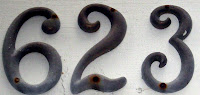A Uranus-Neptune conjunction in Virgo occurred in the year 623.

## Monday, March 15, 2010

### 205

205 = 5 x 41.

205 has two representations as a sum of two squares: 205 = 32 + 142 = 62 + 132.

205 is the hypotenuse of two primitive Pythagorean triples: 2052 = 842 + 1872 = 1332 + 1562.

Every number greater than 205 is the sum of distinct primes of the form 6n + 1.

205 is the number of pairs of twin primes less than 10,000.The Peugeot 205 is a supermini produced by the French car manufacturer Peugeot between 1983 and 1997.

## Friday, March 12, 2010

### 3.14159...

3.14159... (π or pi) is the ratio of a circle's circumference to its diameter.

π is the area of a unit circle. It is also the ratio of the circumference of any convex curve of constant diameter to its diameter (Barbier's theorem).

π is an irrational number and a transcendental number.

22/7 is the best approximation to π, using the ratio of two numbers less than 100.In 1949, the ENIAC calculated π to 2037 places in 70 hours, without making any mistakes. The current record is nearly 2.7 trillion decimal digits, confirmed in January 2010.

Source: Wikipedia

## Thursday, March 11, 2010

### 535

535 = 5 x 107

5, 3, and 5 are the eighth, ninth, and tenth decimal digits of pi.

535 is a number that cannot be written as a sum of three squares.

535535 + (535 - 1) is a prime number.

535 is the smallest palindrome such that its cube can be written as a sum of three prime cubes: 5353 = 3493 + 3793 + 3833.The Indonesian volcano Krakatoa erupted in the year 535.

## Wednesday, March 10, 2010

### 53

53 is a prime number. It is also a Sophie Germain prime because 2 x 53 + 1 = 107, which is also a prime.

5 and 3 are the eighth and ninth decimal digits of pi.

53 has a representation as a sum of two squares: 53 = 22 + 72.

53 is the hypotenuse of a primitive Pythagorean triple: 532 = 282 + 452.

53 is the sum of five consecutive primes: 5 + 7 + 11 + 13 + 17 = 53.The chemical element iodine has atomic number
53.

## Tuesday, March 9, 2010

### 265

265 = 5 x 53.

2, 6, and 5 are the sixth, seventh, and eighth decimal digits of pi.

265 has two representations as a sum of two squares: 265 = 32 + 162 = 112 + 122.

265 is the hypotenuse of two primitive Pythagorean triples: 2652 = 232 + 2642 = 962 + 2472.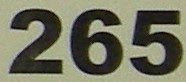Greek mathematician Archimedes, who is studying in Alexandria in the year 265 BC, devised a screw for raising water

Source: Wikipedia